| Technical Writer / Review / Copy Editor: ABCOM Team | Level: Beginner |

# Introduction

Training a deep neural network requires an enormous amount of data. If your machine learning model uses image data, collecting many images becomes a challenge. Consider that you want to detect a Tiger in a picture. Collecting many tiger images would be an undaunting task. So, what we do in such situations is to augment the images that you have to create a larger dataset. Augmentation would mean as simple as rotating the image, shifting it horizontally or vertically, zooming in and out, flipping the image, etc. For a machine learning algorithm, each image is independent and has no correlation with any other image in the set. That is how you can create a large training dataset for your ML development. Though the augmentation may sound like an arduous task, fortunately for us, the Keras library provides us with a simple, yet powerful feature of image augmentation.

In this tutorial you will learn how to perform image augmentation using Keras library. Especially, you will learn how to perform the following types of augmentation.

• Rotation
• Width and height shift
• Brightness
• Shear transformation
• Zoom
• Channel shift
• Flip

# Creating Project

Create a new Google Colab project and rename it to Image_Augmentation. If you are new to Colab, then check out this short video tutorial on Google Colab.

## Import libraries

We will perform image augmentation using `ImageDataGenerator` class in keras library. Starting with TF 2.0, keras is fully integrated with tf, so you do not need to import keras explicitly. Here are all the required imports.

``````%matplotlib inline
import os
import numpy as np
import tensorflow as tf
import shutil
from PIL import Image
from matplotlib import pyplot as plt
``````

I have uploaded a sample image in our GitHub repository. You will use `wget` command to download this into your project. You will learn various image augmentation techniques on this image.

``````!wget 'https://raw.githubusercontent.com/abcom-mltutorials/images/main/cat.jpg'
``````

You can display the image on your screen by using the following code:

``````from IPython import display
display.Image("/content/cat.jpg")
``````

The image is shown here for your quick reference: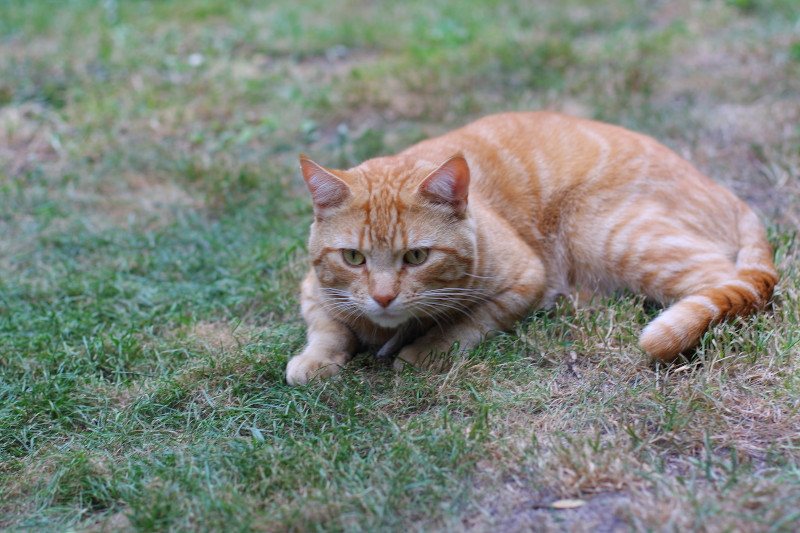Keras library requires you to keep this image in a certain path on your Google Drive. First mount the drive into your Colab project using following code:

``````from google.colab import drive
drive.mount('/content/drive')
``````

You will need to enter the authorization code as instructed on your screen. After the drive is successfully mounted, use the following code to create the required path on your drive and move the image file to the new location.

``````os.mkdir('images')
os.mkdir('images/train')
os.mkdir('images/train/cat')
shutil.move('/content/cat.jpg', 'images/train/cat/cat.jpg')
``````

Note that if the path already exists on your drive, you will need to omit the corresponding mkdir commands from the above code. After the file is successfully moved, you are ready to try out augmentation.

# Image Augmentation

To perform image augmentation we will use keras `ImageDataGenerator` class. When you create an instance of this class, you will set its function parameter to the desired type of augmentation.

First, let me show you how to rotate an image by a certain angle which is selected at random.

## Rotation

To rotate the image, you pass the `rotation_range` parameter to the class constructor.

``````generator = tf.keras.preprocessing.image.ImageDataGenerator(
rotation_range=40)
``````

We can specify the rotation angle as a value between 0 and 360. When you specify a value of 40, as in the above example, the rotation would be done anywhere between 0 and 40. It selects the angle at random on every run. The value closest to the specified maximum is generated.

You perform the rotation using the following code:

``````x, y = next(generator.flow_from_directory('images', batch_size=1))
plt.imshow(x.astype('uint8'));
``````

After rotation we display the image. Here is the output: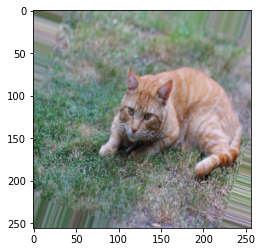Try executing the code a couple of times. Every time, the rotation would be different. Here are a few sample runs in my case.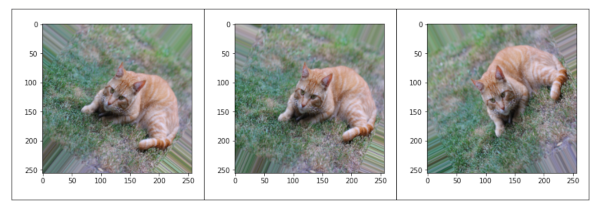## Width and Height Shift

You can shift the image horizontally or vertically to augment it. The following code would shift it horizontally by max 30% and vertically by max 20%.

``````generator= tf.keras.preprocessing.image.ImageDataGenerator(width_shift_range=0.3, height_shift_range=0.2)
x, y = next(generator.flow_from_directory('images', batch_size=1))
plt.imshow(x.astype('uint8'))
``````

We may specify the range as a float or an integer. A float value shows a percentage change, while the integer value would specify the number of pixels by which we desire a shift.

Here are a few examples of the horizontal and vertical shift.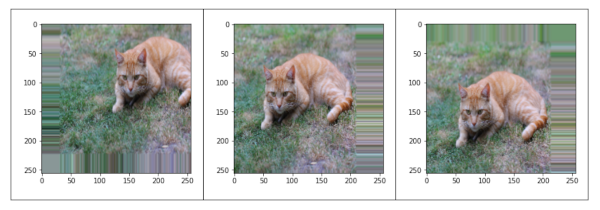## Brightness

The lighting conditions at the time of capturing the image may be a dim or too bright light. Vary the brightness of the image can simulate pictures under different lighting conditions. We change the image brightness by specifying the `brighness_range` parameter. The following code sets the brightness range between 0.6 and 2.0.

``````generator = tf.keras.preprocessing.image.ImageDataGenerator(
brightness_range=(0.6, 2.0)
)
x, y = next(generator.flow_from_directory('images', batch_size=1))
plt.imshow(x.astype('uint8'));
``````

Here are a few samples with varying brightness.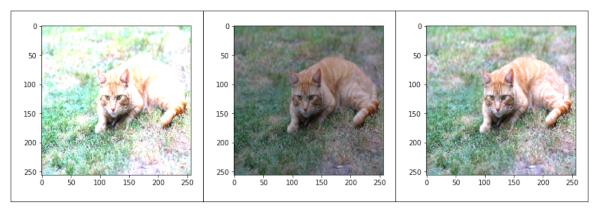## Shear Transformation

The shear transformation slants the image. This creates a sort of ‘stretch’ in the image, which we do not see in rotation. The `shear_range` specifies the angle of the slant in degrees.

``````generator = tf.keras.preprocessing.image.ImageDataGenerator(
shear_range=60
)

x, y = next(generator.flow_from_directory('images', batch_size=1))
plt.imshow(x.astype('uint8'));
``````

Here are a few samples of shear transformations: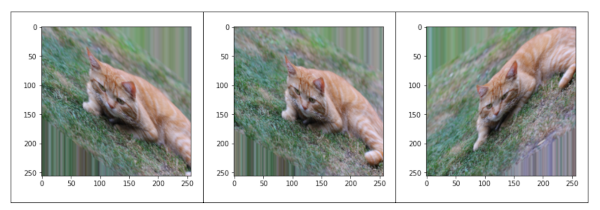## Zooming in-out

This transformation can randomly zoom in or zoom out of the image. It takes two float values, upper and lower limits. Any value smaller than 1 will zoom in on the image. Whereas any value greater than 1 will zoom out on the image.

``````generator = tf.keras.preprocessing.image.ImageDataGenerator(
zoom_range=[0.5, 1.5]
)

x, y = next(generator.flow_from_directory('images', batch_size=1))
plt.imshow(x.astype('uint8'))
``````

Here are a few samples showing the effect of zooming-in and zooming-out.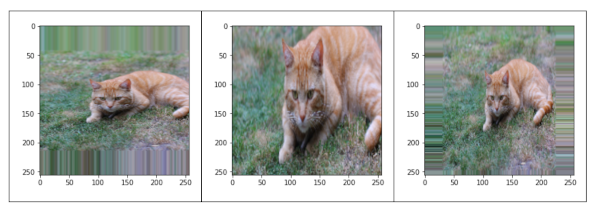## Channel Shift

This transformation changes the channel values at random. You will specify the range for a channel shift in the parameter value. The following code accomplishes this:

``````generator = tf.keras.preprocessing.image.ImageDataGenerator(
channel_shift_range=100 #-100 to 100
)

x, y = next(generator.flow_from_directory('images', batch_size=1))
plt.imshow(x.astype('uint8'));
``````

Here are a few samples: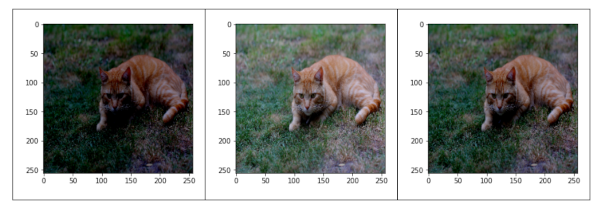# Flip

With this transformation, you will flip the image horizontally or vertically. This code accomplishes this:

``````generator = tf.keras.preprocessing.image.ImageDataGenerator(
horizontal_flip=True,
vertical_flip=True
)

x, y = next(generator.flow_from_directory('images', batch_size=1))
plt.imshow(x.astype('uint8'));
``````

Here are a few samples: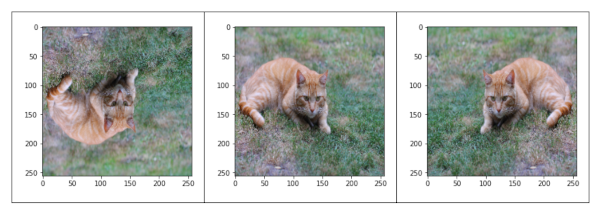# Summary:

Machine learning model training typically requires a very large dataset. Collecting a huge dataset for training the models on image data is thus an enormous challenge, as usually it is practically impossible to click so many pictures of a single subject. So, we use image augmentation techniques for creating additional images. We take an existing actual image and transform it into various images by image augmentation techniques. Keras provides a ready-to-use class called ImageGenerator for performing such transformations. In this tutorial, you learned to use this class for performing transformations such as rotating, horizontal and vertical shift, changing brightness, shear, zooming in-n-out, channel shift and flipping horizontally or vertically. Use it practically in your next ML project to inflate your image dataset.